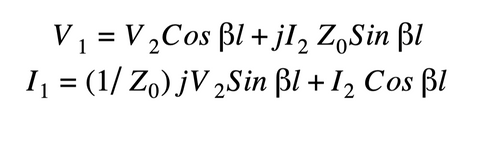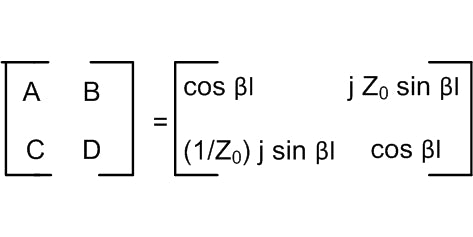# ABCD Parameters of Transmission Lines

### Key Takeaways

• The ABCD parameters of a transmission line give the relationship of the input voltage and currents to the output voltage and currents.
• ABCD parameters simplify complex calculations when transmission lines are cascaded.
• ABCD parameters are dependent on the length of a transmission line.Modeling a system as a two-port network is common in electronics circuit analysis. Transmission line characterization can be done using two-port network models such as hybrid parameters,scattering,parameters, impedance parameters, admittance parameters, or ABCD parameters (also known as transmission parameters, chain parameters, or cascade parameters).

Two-port models make the analysis of transmission lines easier and the relationship between the port voltages and currents of interest can be deduced. Among the parameters, the ABCD parameters of a transmission line give the relationship of the input voltage and currents to the output voltage and currents. In this article, we will take a closer look at the two-port model of a transmission line and its ABCD parameters.

## ABCD Parameters of a Transmission Line

To characterize transmission lines using ABCD parameters, the line is modeled as a general two-port network.

The two-port model of the transmission line takes input current I1 at port 1, with an input voltage equal to V1. The output voltage and current are V2 and I2, respectively. The current directions are taken so that I1  is entering and I2 is leaving the two-port network. Among the four electrical quantities, V1 and I1 are the dependent variables. The voltage V1 and current I1 are represented mathematically in terms of independent variables V2 and I as follows:The same can be represented in matrix form as:ABCD parameters simplify complex calculations when transmission lines are cascaded. The cascaded connection can be modeled as a single two-port network having ABCD parameters equal to the product of ABCD parameters of each individual transmission line in the cascade.

The table below gives more details on ABCD parameters:

 Parameter Ratio Unit A Open circuit reverse voltage transfer ratio Unitless B Short circuit reverse transfer impedance Ohms C Open circuit reverse transfer admittance Siemens D Short circuit reverse current transfer ratio Unitless

ABCD parameters

To model a two-port network of transmission lines, we assume that the network is linear and bilateral. The type of network can be identified from ABCD parameters.

 Type of Circuit ABCD Parameter Values or Relationship Symmetrical A=D Reciprocal AD-BC=1 Open-circuited A=C=0 Short-circuited B=D=0

Conditions of ABCD parameters

## ABCD Parameters of Lossless Transmission Lines

Consider a lossless tranmission line of uniform length. In this line, the attenuation constant 𝛼ᶛ is equal to zero and the phase constant is given by 𝛽. The voltages and currents of the lossless transmission line can be given as follows:Comparing the equations above with the first two equations in this article, the ABCD parameters of a lossless transmission line can be given in matrix form as:From the matrix above, it is clear that the ABCD parameters are dependent on the length of a transmission line; therefore, the ABCD parameters of a short line vs. a  long transmission line will differ from each other.

Understanding the ABCD parameters of transmission lines is useful for analyzing the characteristics and behaviors of single and multiple line structures. Cadence’s software can also help with transmission line modeling and analysis.